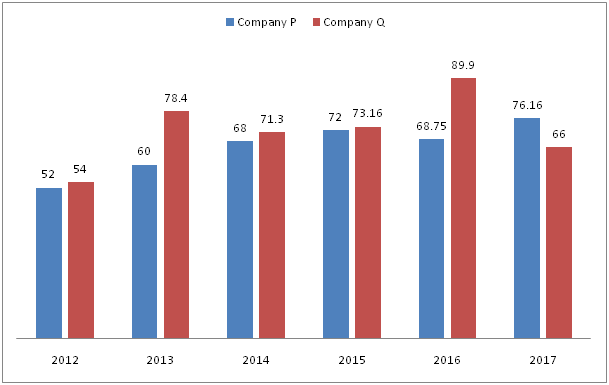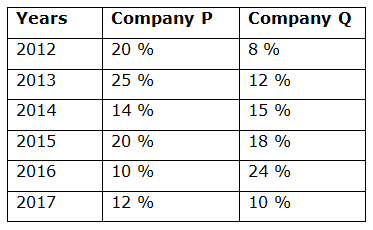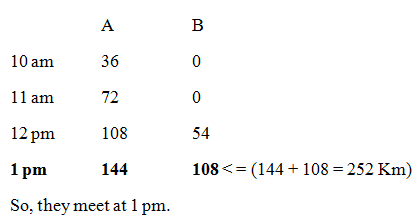# CWC/FCI Prelims 2019 – Quantitative Aptitude Questions (Day-13)

Dear Readers, Exam Race for the Year 2019 has already started, To enrich your preparation here we are providing new series of Practice Questions on Quantitative Aptitude – Section for CWC/FCI Exam. Aspirants, practice these questions on a regular basis to improve your score in aptitude section. Start your effective preparation from the right beginning to get success in upcoming CWC/FCI Exam.

[WpProQuiz 5524]

1) Two stations A and B are 252 km apart on a straight line. One train starts from A at 9 a.m. and travels towards B at 36 km/hr. Another train starts from B at 11 a.m. and travels towards A at a speed of 54 km/hr. At what time will they meet?

a) 2 pm

b) 1.30 pm

c) 1 pm

d) 2.30 pm

e) None of these

2) A shopkeeper sold a book at 25 % discount on the marked price but he gains 10 %. If the marked price of the book is Rs. 550, then find the cost price of the book?

a) Rs. 375

b) Rs. 420

c) Rs. 435

d) Rs. 450

e) None of these

3) The average age of 28 students in a class is 17 years. If the age of the teacher is included then the average increases by 1 then find the age of the teacher

a) 51 years

b) 53 years

c) 48 years

d) 46 years

e) None of these

4) A can do a work in 15 days, B can do it in 12 days but C can do (3/4)th of the work in 18 days. Find the time taken by all together to complete the work?

a) 4 (7/15) days

b) 5 (5/23) days

c) 6 (3/19) days

d) 4 (13/25) days

e) None of these

5) P, Q and R started a business by investing Rs. 32000, Rs. 25000 and Rs. 40000 respectively. After 5 months, Q invested Rs. 5000 more but R withdraw Rs. 8000. Find the share of P, if the share of R at the end of the year is Rs. 63600?

a) Rs. 55200

b) Rs. 48500

c) Rs. 57600

d) Rs. 51300

e) None of these

Directions (Q. 6 – 10): Study the following information carefully and answer the given questions:

The following bar graph shows the Income (In lakhs) of two different companies over the years.The table below shows the profit % of company P and company Q over the years.Note:

Profit % = [(Income – Expenditure)/Expenditure]*100

6) Find the total Income of company Q in all the given years together?

a) 516.28 lakhs

b) 432.76 lakhs

c) 458.34 lakhs

d) 524.52 lakhs

e) None of these

7) The total income of company P in the year 2012, 2014 and 2016 together is approximately what percentage of the total income of company Q in the year 2013, 2015 and 2017 together?

a) 72 %

b) 64 %

c) 102 %

d) 88 %

e) 115 %

8) Find the difference between the total expenditure of company P in the year 2013 and 2015 together to that of total expenditure of company Q in the year 2012 and 2014 together?

a) 4 lakhs

b) 8 lakhs

c) 10 lakhs

d) 12 lakhs

e) None of these

9) Total expenditure of company P and Q together in the year 2015 is approximately what percentage of total expenditure of company P and Q together in the year 2017?

a) 118 %

b) 73 %

c) 95 %

d) 58 %

e) 125 %

10) Find the ratio between the total expenditure of both the companies together in the year 2013 to that of the total expenditure of both the companies together in the year 2016?

a) 56: 77

b) 23: 34

c) 7: 13

d) 118: 135

e) None of these

They meet x hours after 9 a.m.

Distance covered by A in x hours = 36x km

Distance covered by B in (x – 2) hours = 54(x – 2) km

36x + 54(x – 2) = 252

36x + 54x = 252 + 108

90x = 360

X = 4

They meet at 1 p.m.

(Or)Marked price*[(100 – Discount %)/100] = Cost price*[(100 + Gain %)/100]

550*(75/100) = CP*(110/100)

CP = 550*(75/100)*(100/110)

Cost price of the book = Rs. 375

Total age of 28 students = 28*17 = 476

Total age of 28 students and teacher = 29*18 = 522

Age of teacher = 522 – 476 = 46 years

Shortcut:

N       Average

28      17

29      18

If both n and Average increased by 1, then the age of the teacher is,

Age of teacher = (28 + 18) or (29 + 17) = 46 years

A’s one day work = 1/15

B’s one day work = 1/12

C can do the whole work in, = 18*(4/3) = 24 days

C’s one day work = 1/24

(A + B + C)’s one day work = (1/15) + (1/12) + (1/24)

= > (8 + 10 + 5)/120

= > 23/120

A, B and C can complete the whole work in, = 120/23 = 5 (5/23) days

The share of P, Q and R

= > [32000*12]: [25000*5 + 30000*7]: [40000*5 + 32000*7]

= > 384000: (125000 + 210000): (200000 + 224000)

= > 384000: 335000: 424000

= > 384: 335: 424

The share of R = 63600

424’s = 63600

1’s = 63600/424 = 150

The share of P = 384’s = Rs. 57600

Direction (6-10) :

The total Income of company Q in all the given years together

= > 54 + 78.4 + 71.3 + 73.16 + 89.9 + 66

= > 432.76 lakhs

The total income of company P in the year 2012, 2014 and 2016 together

= > 52 + 68 + 68.75 = 188.75 lakhs

The total income of company Q in the year 2013, 2015 and 2017 together

= > 78.4 + 71.3 + 66 = 215.7 lakhs

Required % = (188.75/215.7)*100 = 87.505 % = 88 %

The total expenditure of company P in the year 2013 and 2015 together

= > 60*(100/125) + 72*(100/120)

= > 48 + 60 = 108 lakhs

The total expenditure of company Q in the year 2012 and 2014 together

= > 54*(100/108) + 71.3*(100/115)

= > 50 + 62 = 112 lakhs

Required difference = 112 – 108 = 4 lakhs

Total expenditure of company P and Q together in the year 2015

= > 72*(100/120) + 73.16*(100/118)

= > 60 + 62 = 122 lakhs

Total expenditure of company P and Q together in the year 2017

= > 76.16*(100/112) + 66*(100/110)

= > 68 + 60 = 128 lakhs

Required % = (122/128)*100 = 95 %

The total expenditure of both the companies together in the year 2013

= > 60*(100/125) + 78.4*(100/112)

= > 48 + 70 = 118 lakhs

The total expenditure of both the companies together in the year 2016

= > 68.75*(100/110) + 89.9*(100/124)

= > 62.5 + 72.5 = 135 lakhs

Required ratio = 118: 135